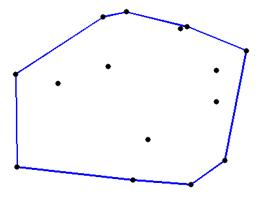favorite We need a little bit of your help to keep things running, click on this banner to learn more
Competitions

# Convex HullFinding the convex hull of a set of points is an important problem that is often part of a larger problem. There are many algorithms for finding the convex hull. Since problems involving the convex hull sometimes appear in the ACM World Finals, it is a good idea for contestants to know some of these algorithms.

Finding the convex hull of a set of points in the plane can be divided into two sub-tasks. First, given a set of points, find a subset of those points that, when joined with line segments, form a convex polygon that encloses all of the original points. Second, output the points of the convex hull in order, walking counter-clockwise around the polygon. In this problem, the first sub-task has already been done for you, and your program should complete the second sub-task. That is, given the points that are known to lie on the convex hull, output them in order walking counter-clockwise around the hull.

Input

The first line of input contains a single integer 3n100000, the number of points. The following n lines of input each describe a point. Each of these lines contains two integers and either a Y or an N, separated by spaces. The two integers specify the x- and y-coordinates of the point. A Y indicates that the point is on the convex hull of all the points, and a N indicates that it is not. The x- and y-coordinates of each point will be no less than -1000000000 and no greater than 1000000000. No point will appear more than once in the input. The points in the input will never all lie on a line.

Output

First, output a line containing a single integer m, the number of points on the convex hull. Next output m lines, each describing a point on the convex hull, in counter-clockwise order around the hull. Each of these lines should contain the x-coordinate of the point, followed by a space, followed by the y-coordinate of the point. Start with the point on the hull whose x-coordinate is minimal. If there are multiple such points, start with the one whose y-coordinate is minimal.

Time limit 1 second
Memory limit 64 MiB
Input example #1
```5
1 1 Y
1 -1 Y
0 0 N
-1 -1 Y
-1 1 Y
```
Output example #1
```4
-1 -1
1 -1
1 1
-1 1
```